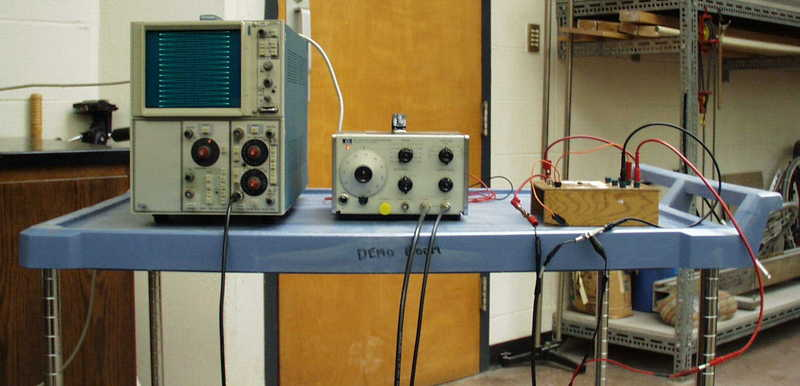# AC CIRCUITS

5L10.10 EFFECT OF IMPEDANCE ON CURRENT
5L20.10 PHASE IN AC CIRCUITS
5L20.20 RESONANCE IN AC CIRCUITS

 5L10.10 Effect of Changing Impedance on Current Show the effect of inductance on "AC resistance"  (impedance). A flashlight bulb is connected in series with the jumping ring coil . A Variac is used to supply ~ 6v AC. The brightness of the bulb will vary as you move the iron core in and out of the coil.Setup Requirements: Assembled as needed. Start with Variac on zero.   Equations: Z2 = R2 + (wL)2   Safety Issues: AC voltage present at bare terminals. Limit voltage to 6v to avoid burning out flashlight bulb or 10v if using car bulb.

 5L20.10 Phase in AC Circuit Hook up 2 channel oscilloscope to show that voltage across a capacitor is not in phase with the total voltage in a RLC circuit. Moving a rod in and out of coil will show how changing inductance affects phase. Frequency can be changed.Setup Requirements: Assembled as needed. One day advance notice needed.   Equations: Phase angle between total voltage and capacitor voltage depends on resistance, capacitance and inductance.   Safety Issues: None specific to this demonstration

 5L20.20 Resonance in AC Circuit Demonstrate resonance in LRC circuit with AC source. The amplitude of the output voltage shown on the oscilloscope varies as the frequency of the input voltage is varied.Setup Requirements: Assembled as needed. Ask 1 day ahead of time. Using coil and resistor on PASCO board and a .05 mfd capacitor gives a narrow peak at a frequency of ~ 8,000 Hz. Set up to measure capacitor voltage. If available use oscilloscope with frequency meter.   Equations: Amplitude is largest when frequency of AC source equals resonant frequency of LC combination.   Safety Issues: None specific to this demonstration